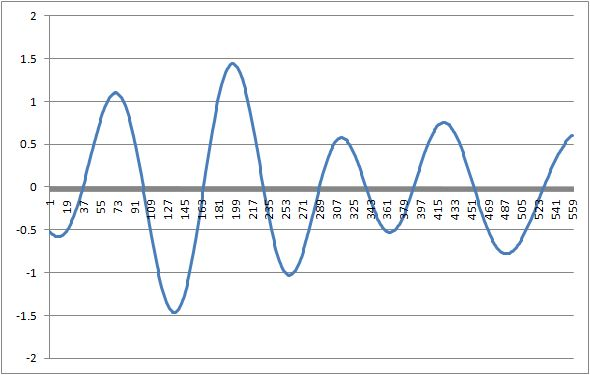# Curve Analysis

The methods related to curve analysis assume that the underlying signal is quasi-sinusoidal or quasi-periodic. These methods constitute a kernel of methods that will be further expanded in future releases to analyze quasi-periodic waves and signals.

## Peaks and Bottoms

These methods compute respectively the number of peaks and the number of bottoms in the time-series.

## Curve Similarity

Curve similarity computes an index of similarity that is not the “simple” mean squared difference between the two curves and is computed on the first derivative of the signal. The lower the index the more similar the two curves, the higher the more different they are. The index is always positive.

## Average Period

The method computes the average period of the signal that is supposed to be quasi-sinusoidal. The computation is useful when there are more than three periods in the signal, for less than three periods the average is not statistically significant.

## Average Period Deviation

The simple measure of average period is not enough to analyze quasi-sinusoidal time-series. This method computes the average deviation from the mean period of the signal.

This is an example of a quasi-periodic signal:Its average period is 119.75 and its average period deviation is 5.875. This nearly 5% variation in the period of the quasi-sinusoid and its average period are very important information that allows us to compute a reliable forecast.

 Forecasting Methods Holt Winter’s, Series Decomposition and Wavelet Benchmarks Time Series Forecasting Use of the Moving Average in Time-series Forecasting Forecasting Concepts Denoising Techniques Error Statistics Computational Performance Fast Fourier Transform Moving Averages Kernel Smoothing Active Moving Average Savitsky-Golay Smoothing Fractal Projection Downloading Financial Data from Yahoo Multiple Regression Digital Signal Processing Principal Component Analysis Curve Analysis Options Pricing with Black-Scholes Markowitz Optimal Portfolio Time-series preprocessing